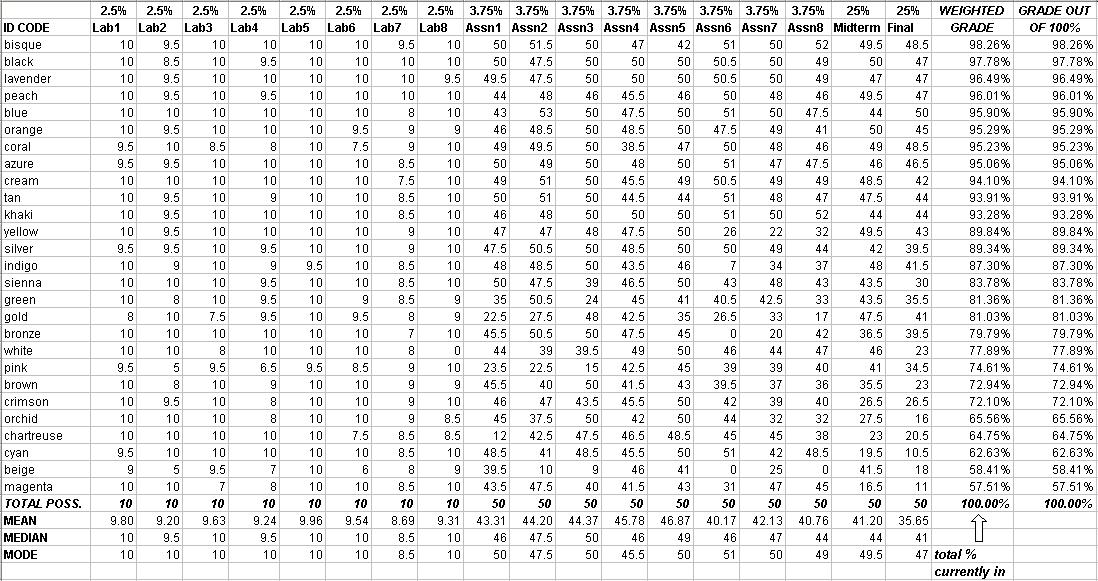# ESc 014 Spring 2002 Final Grades

Intangibles like effort, improvement, and voluntary participation were considered for borderline cases only. "Borderline" means approximately 0.1-0.2% away from the next grade.

### Explanation of statistics:

Mean: the average
Median: the "middle" score (for our class of 27, 14 are at or above the median score and 14 are at or below the median score.)
Mode: the most common score

• 93+ = A
• 90 - 92.99 = A-
• 87 - 89.99 = B+
• 83 - 86.99 = B
• 80 - 82.99 = B-
• 77 - 79.99 = C+
• 73 - 76.99 = C
• 70 - 72.99 = C-
• 60 - 69.99 = D
• -59.99 = F
• 93+ = A
• 90 - 92.99 = A-
• 87 - 89.99 = B+
• 83 - 86.99 = B
• 80 - 82.99 = B-
• 70 - 79.99 = C
• -69.99 = FESc 014 homepage